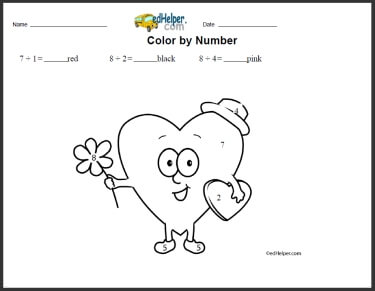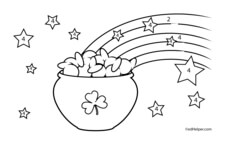Worksheets and No Prep Teaching Resources
Math Worksheets

# Color by Division Worksheets

Learning division math facts can be a challenge, but not when combined with the engaging activity of coloring sheets! These ingenious worksheets will help students effortlessly learn basic math while creating a striking piece of art. Students will want to finish the lively picture and will quickly master all division facts that are so vital for future math success!Math Lesson: Color by Numbers - February Color by Number BookDivision Coloring: a division equation given for each color Multiplication and Division Coloring: a multiplication or division equation given for each color

Math Lesson: Color by Numbers - March Color by Number BookDivision Coloring: a division equation given for each color Multiplication and Division Coloring: a multiplication or division equation given for each color

Math Lesson: Color by Numbers - August Color by Number BookDivision Coloring: a division equation given for each color Multiplication and Division Coloring: a multiplication or division equation given for each color

Have a suggestion or would like to leave feedback?